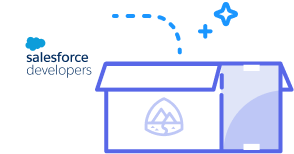function readOnly(count){ }Starting November 20, the site will be set to read-only. On December 4, 2023,
forum discussions will move to the Trailblazer Community.
ShowAll Questionssorted byDate PostedMMA_FORCE

I have to find out the total students for every grade and term level...

So I am doing a count for every grade level and placing the value in separate strings and then upsert those valued to the object.

For example:

numStudents10 = [select count() from Contact where AccountId = :schoolId and Grade__c=10 ]; numStudents11 = [select count() from Contact where AccountId = :schoolId and Grade__c=11 ]; numStudents12 = [select count() from Contact where AccountId = :schoolId and Grade__c=12 ]; numStudents9 = [select count() from Contact where AccountId = :schoolId and Grade__c=9 ];

Then I have to do separate for terms Like: For all grades....

numStudents10_1 = [select count() from Contact where AccountId = :schoolId and Grade__c=10 and Term__c=10.1 ]; numStudents10_2 = [select count() from Contact where AccountId = :schoolId and Grade__c=10 and Term__c=10.2 ]; numStudents10_3 = [select count() from Contact where AccountId = :schoolId and Grade__c=10 and Term__c=10.3 ]; numStudents10_4 = [select count() from Contact where AccountId = :schoolId and Grade__c=10 and Term__c=10.4 ];

Does anyone have a better way to do this??

ThanksBest Answer chosen by Admin (Salesforce Developers)prageeth

Hello

You can't dynamicaly create variable names such as "numStudents + i".

However you can simplify your code if you like to use a Map instead of variables.

Then your controller would be something like below.

Here I assume your "Grade__c" field is a number field with no decimal places and "Term__c" is a number field with one decimal place.

public class MyController{

Map<String, Integer> myMap = new Map<String, Integer>();

Id schoolId = '001A0000007C0So';//change this value as necessary

public void setData(){

for (integer i = 9; i<13; i++) {

Integer itemCount = [select count() from Contact where

AccountId = :schoolId

];

myMap.put('numStudents' + i, itemCount);

for (Double j = 1; j <= 4; j++) {

itemCount = [select count() from Contact where

AccountId = :schoolId

and Term__c=:(i + j/10)

];

myMap.put('numStudents' + i + '_' + j.intValue(), itemCount);

}

}

}

}

You can call the setData() method to fill the Map with data.

When you want to get a certain value from the Map, you can use a method like this.

public Integer getValue() {

return myMap.get('numStudents10_1');

}

If this is not clear for you I am ready to explain it further.CaptainObvious

You may be able to simplify the SOQL with the Spring '10 release...

select Grade__c, Term__c, count() cnt from Contact where AccountId =:schoolId group by rollup(grade__c, term__c)

See the example here.

Message Edited by CaptainObvious on 02-24-2010 10:10 AMMMA_FORCE

Thanks But I was thinking of doing this but do not know how to change value in for loop...

for(integer i = 9; i<13; i++){ numStudents+i = [select count() from Contact where AccountId = :schoolId and Grade__c=+'i' ]; }

I did try the aggregate method but was getting errors: Kept getting error:

Error: Compile Error: Grouped field should not be aggregated: Grade__c at line 77 column 22

List<Contact> numStudents90; numStudents90 = [select Grade__c, count(Grade__c) cnt from Contact where AccountId = :schoolId GROUP BY ROLLUP(Grade__c) ];

Coolprageeth

Hello

You can't dynamicaly create variable names such as "numStudents + i".

However you can simplify your code if you like to use a Map instead of variables.

Then your controller would be something like below.

Here I assume your "Grade__c" field is a number field with no decimal places and "Term__c" is a number field with one decimal place.

public class MyController{

Map<String, Integer> myMap = new Map<String, Integer>();

Id schoolId = '001A0000007C0So';//change this value as necessary

public void setData(){

for (integer i = 9; i<13; i++) {

Integer itemCount = [select count() from Contact where

AccountId = :schoolId

];

myMap.put('numStudents' + i, itemCount);

for (Double j = 1; j <= 4; j++) {

itemCount = [select count() from Contact where

AccountId = :schoolId

and Term__c=:(i + j/10)

];

myMap.put('numStudents' + i + '_' + j.intValue(), itemCount);

}

}

}

}

You can call the setData() method to fill the Map with data.

When you want to get a certain value from the Map, you can use a method like this.

public Integer getValue() {

return myMap.get('numStudents10_1');

}

If this is not clear for you I am ready to explain it further.

This was selected as the best answerMMA_FORCE

Very clear Thank you...

I did try to do it in a Aggregate Function such as Rollup...

I tried this:

// Get all the data in one query AggregateResult[] groupedResults = [select Term__c, count(Student__r.Name) cnt from Summary__c where Student__r.AccountId = :schoolId GROUP BY RollUp(Term__c)]; for (AggregateResult ar : groupedResults) { //items.add(new c(String.ValueOf(ar.get('Term__c')), String.ValueOf(ar.get('cnt')))); // numStudentsT.put(String.ValueOf(ar.get('Term__c')),ar.get('cnt')); c.put(String.ValueOf(ar.get('Term__c')),ar.get('cnt')); }

AND This:

Map<String, Integer> numStudentsT = new Map<String, Integer>();

// Get all the data in one query AggregateResult[] groupedResults = [select Term__c, count(Student__r.Name) cnt from Summary__c where Student__r.AccountId = :schoolId GROUP BY RollUp(Term__c)]; for (AggregateResult ar : groupedResults) { //items.add(new c(String.ValueOf(ar.get('Term__c')), String.ValueOf(ar.get('cnt')))); numStudentsT.put(String.ValueOf(ar.get('Term__c')),ar.get('cnt')); //c.put(String.ValueOf(ar.get('Term__c')),ar.get('cnt')); }

Would you know what am I doing incorrect here... I thought this would be easier then the other... But it does not seem like it...